## How to Calculate and Solve for Shortest Distance Between Two Points | Latitude and Longitude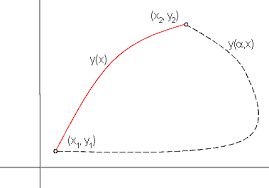The image above represents shortest distance between two points.

To compute for shortest distance between two points, two essential parameters are needed and these parameters are Value of R and Angle Subtended at the Centre by the Chord (A(2β)).

The formula for calculating the shortest distance between two points:

y = (A(2β) / 360) x 2πR

Where;

y = Shortest Distance Between Two Points
A(2β) = Angle Subtended at the Centre by the Chord
R = Radius of the Earth

Let’s solve an example;
Find the shortest distance between two points with the angle subtended at the centre by the chord as 40 and radius of the earth as 6400.

This implies that;

A(2β) = Angle Subtended at the Centre by the Chord = 40°
R = Radius of the Earth = 6400

y = (A(2β) / 360) x 2πR
y = (40 / 360) x 2π x 6400
y = 0.111 x 2π x 6400
y = 0.111 x 40212.38
y = 4468.04

Therefore, the shortest distance between two points is 4468.04 km.

## How to Calculate and Solve for Part Shrinkage Ratio | Polymer & Textile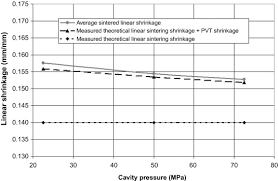The image above represents part shrinkage ratio.

To compute for part shrinkage ratio, two essential parameters are needed and these parameters are mold dimension (DM) and part dimension (DP).

The formula for calculating part shrinkage ratio:

RPS = DM – DP / DM

Where:

RPS = Part Shrinkage Ratio
DM = Mold Dimension
DP = Part Dimension

Let’s solve an example;
Find the part shrinkage ratio when the mold dimension is 8 and the part dimension is 10.

This implies that;

DM = Mold Dimension = 8
DP = Part Dimension = 10

RPS = DM – DP / DM
RPS = 8 – 10 / 8
RPS = -2 / 8
RPS = -0.25

Therefore, the part shrinkage ratio is -0.25.

## How to Calculate and Solve for Degree of Polymerization | Both Mechanisms | Polymer & Textile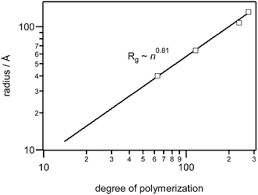The image above represents degree of polymerization | both mechanisms.

To compute for degree of polymerization, two essential parameters are needed and these parameters are parameter (σ) and parameter (V).

The formula for calculating the degree of polymerization:

Xn = 2V / 1 + σ

Where:

Xn = Degree of Polymerization
σ = Parameter
V = Parameter

Let’s solve an example;
Find the degree of polymerization when the parameter is 12 and the parameter is 18.

This implies that;

σ = Parameter = 12
V = Parameter = 18

Xn = 2V / 1 + σ
Xn = 2(18) / 1 + 12
Xn = 36 / 13
Xn = 2.769

Therefore, the degree of polymerization is 2.769.

Calculating the Parameter when the Degree of Polymerization and Parameter is Given.

V = Xn (1 + σ) / 2

Where:

V = Parameter
σ = Parameter
Xn = Degree of Polymerization

Let’s solve an example;
Find the parameter when the degree of polymerization is 22 and the parameter is 4.

This implies that;

Xn = Degree of Polymerization = 22
σ = Parameter = 4

V = Xn (1 + σ) / 2
V = 22 (1 + 4) / 2
V = 22 (5) / 2
V = 110 / 2
V = 55

Therefore, the parameter is 55.

## How to Calculate and Solve for Free Volume of Polymer | Polymer & Textile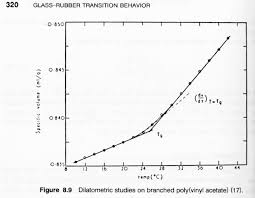The image above represents free volume of polymer.

To compute for free volume of polymer, two essential parameters are needed and these parameters are specific volume (V) and volume of solidly packed molecules (Vs).

The formula for calculating free volume of polymer:

Vf = V – Vs

Where:

Vf = Free Volume of Polymers
V = Specific Volume
Vs = Volume of Solidly Packed Molecules

Let’s solve an example;
Find the free volume of polymer when the specific volume is 30 and the volume of solidly packed molecules is 10.

This implies that;

V = Specific Volume = 30
Vs = Volume of Solidly Packed Molecules = 10

Vf = V – Vs
Vf = 30 – 10
Vf = 20

Therefore, the free volume of the polymer is 20.

Calculating the Specific Volume when the Free Volume of Polymer and the Volume of Solidly Packed Molecules.

V = Vf + Vs

Where;

V = Specific Volume
Vf = Free Volume of Polymers
Vs = Volume of Solidly Packed Molecules

Let’s solve an example;
Find the specific volume when the free volume of polymer is 24 and the volume of solidly packed molecules is 12.

This implies that;

Vf = Free Volume of Polymers = 24
Vs = Volume of Solidly Packed Molecules = 12

V = Vf + Vs
V = 24 + 12
V = 36

Therefore, the specific volume is 36.

## How to Calculate and Solve for TEX | Polymer & Textile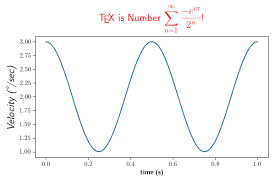The image above represents TEX.

To compute for TEX, two essential parameters are needed and these parameters are mass (M) and length (L).

The formula for calculating TEX:

TEX = M/L x 1000

Where:

TEX = TEX
M = Mass
L = Length

Let’s solve an example;
Find the TEX when the mass is 8 and the length is 14.

This implies that;

M = Mass = 8
L = Length = 14

TEX = M/L x 1000
TEX = 8/14 x 1000
TEX = 0.57 x 1000
TEX = 571.4

Therefore, the TEX is 571.4.

Calculating the Mass when the TEX and the Length is Given.

M = TEX x L / 1000

Where:

M = Mass
TEX = TEX
L = Length

Let’s solve an example;
Find the mass when the TEX is 40 and the length is 8.

This implies that;

TEX = TEX = 40
L = Length = 8

M = TEX x L / 1000
M = 40 x 8 / 1000
M = 320 / 1000
M = 0.32

Therefore, the mass is 0.32.

## How to Calculate and Solve for TEX | Denier | Polymer & Textile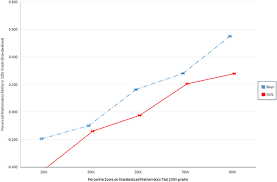The image above represents TEX | Denier.

To compute for TEX | Denier, one essential parameter is needed and this parameter is denier (D).

The formula for calculating the TEX | Denier:

TEX = Denier / 9

Where;

TEX = TEX
D = Denier

Let’s solve an example;
Given that denier is 12. Find the TEX | Denier?

This implies that;

D = Denier = 12

TEX = Denier / 9
TEX = 12 / 9
TEX = 1.33

Therefore, the TEX is 1.33.

## How to Calculate and Solve for Pressure Gradient | Polymer & Textile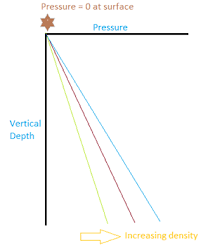The image above represents pressure gradient.

To compute for pressure gradient, four essential parameters are needed and these parameters are value (α), viscosity (μ), screw rotation speed (N) and proportionality constant that depends on screw geometry (B).

The formula for calculating pressure gradient:

ΔP = αμN / B

Where;

α = Value
μ = Viscosity
N = Screw Rotation Speed
B = Proportionality Constant that Depends on Screw Geometry

Let’s solve an example;
Find the pressure gradient when the value is 2, viscosity is 9, screw rotation speed is 20 and proportionality constant that depends on screw geometry is 24.

This implies that;

α = Value = 2
μ = Viscosity = 9
N = Screw Rotation Speed = 20
B = Proportionality Constant that Depends on Screw Geometry = 24

ΔP = αμN / B
ΔP = (2)(9)(20) / 24
ΔP = 360 / 24
ΔP = 15

Therefore, the pressure gradient is 15.

Calculating the Value when the Pressure Gradient, Viscosity, Screw Rotation Speed and Proportionality Constant that Depends on Screw Geometry is Given.

α = ΔP x B / μN

Where;

α = Value
μ = Viscosity
N = Screw Rotation Speed
B = Proportionality Constant that Depends on Screw Geometry

Let’s solve an example;
Find the value when the pressure gradient is 20, viscosity is 5, screw rotation speed is 11 and the proportionality constant that depends on screw geometry is 7.

This implies that;

ΔP = Pressure Gradient = 20
μ = Viscosity = 5
N = Screw Rotation Speed = 11
B = Proportionality Constant that Depends on Screw Geometry = 7

α = ΔP x B / μN
α = 20 x 7 / (5)(11)
α = 140 / 55
α = 2.54

Therefore, the value is 2.54.

## How to Calculate and Solve for Apparent Shear Stress | Slit Die | Polymer & Textile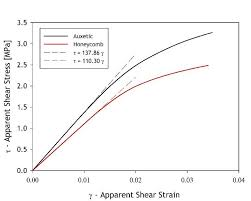The image above represents the apparent shear stress | slit die.

To compute for apparent shear stress | slit die, three essential parameters are needed and these parameters are height of die (h), pressure gradient (ΔP) and flow length (L).

The formula for calculating the apparent shear stress | slit die:

τapp = h . ΔP / 2L

Where:

τapp = Apparent Shear Stress | Slit Die
h = Height of Die
L = Flow Length

Let’s solve an example;
Find the apparent shear stress | slit die when the height of die is 7, pressure gradient is 12 and the flow length is 16.

This implies that;

h = Height of Die = 7
ΔP = Pressure Gradient = 12
L = Flow Length = 16

τapp = h . ΔP / 2L
τapp = 7 . (12) / 2(16)
τapp = 84 / 32
τapp = 2.625

Therefore, the apparent shear stress is 2.625.

Calculating the Height of Die when the Apparent Shear Stress, Pressure Gradient and the Flow Length is Given.

h = τapp x 2L / ΔP

Where:

h = Height of Die
τapp = Apparent Shear Stress | Slit Die
L = Flow Length

Let’s solve an example;
Find the height of die when the apparent shear stress | slit die is 32, the pressure gradient is 10 and the flow length is 8.

This implies that;

τapp = Apparent Shear Stress | Slit Die = 32
ΔP = Pressure Gradient = 10
L = Flow Length = 8

h = τapp x 2L / ΔP
h = 32 x 2 (8) / 10
h = 32 x 16 / 10
h = 512 / 10
h = 51.2

Therefore, the height of die is 51.2.

## How to Calculate and Solve for Plastic Pressure | Polymer & Textile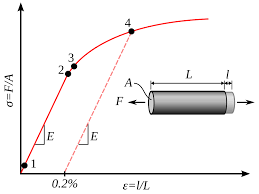The image above represents plastic pressure.

To compute for plastic pressure, two essential parameters are needed and these parameters are hydraulic pressure (PH) and intensification ratio (Ir).

The formula for calculating plastic pressure:

Pp = PH x Ir

Where;

Pp = Plastic Pressure
PH = Hydraulic Pressure
Ir = Intensification Ratio

Let’s solve an example;
Find the plastic pressure when the hydraulic pressure is 20 and the intensification ratio is 44.

This implies that;

PH = Hydraulic Pressure = 20
Ir = Intensification Ratio = 44

Pp = PH x Ir
Pp = 20 x 44
Pp = 880

Therefore, the plastic pressure is 880.

Calculating the Hydraulic Pressure when the Plastic Pressure and the Intensification Ratio is Given.

PH = Pp / Ir

Where;

PH = Hydraulic Pressure
Pp = Plastic Pressure
Ir = Intensification Ratio

Let’s solve an example;
Find the hydraulic pressure when the plastic pressure is 24 and the intensification ratio is 10.

This implies that;

Pp = Plastic Pressure = 24
Ir = Intensification Ratio = 10

PH = Pp / Ir
PH = 24 / 10
PH = 2.4

Therefore, the hydraulic pressure is 2.4.
Continue reading How to Calculate and Solve for Plastic Pressure | Polymer & Textile

## How to Calculate and Solve for Dryer Capacity | Polymer & Textile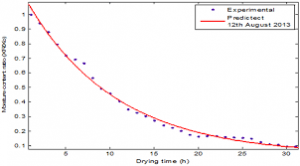The image above represents dryer capacity.

To compute for dryer capacity, two essential parameters are needed and these parameters are material consumption (Mcand drying time (TD).

The formula for calculating dryer capacity:

Dc = Mc / TD

Where:

Dc = Dryer Capacity
Mc = Material Consumption
TD = Drying Time

Let’s solve an example;
Given that the material consumption is 28 and the drying time is 34. Find the dryer capacity?

This implies that;

Mc = Material Consumption = 28
TD = Drying Time = 34

Dc = Mc / TD
Dc = 28 / 34
Dc = 0.82

Therefore, the dryer capacity is 0.82.

Calculating for Material Consumption when the Dryer Capacity and the Drying time is Given.

Mc = Dc x TD

Where:

Mc = Material Consumption
Dc = Dryer Capacity
TD = Drying Time

Let’s solve an example;
Find the material consumption when the dryer capacity is 40 and the drying time is 2.

This implies that;

Dc = Dryer Capacity = 40
TD = Drying Time = 2

Mc = Dc x TD
Mc = 40 x 2
Mc = 80

Therefore, the material consumption is 80.
Continue reading How to Calculate and Solve for Dryer Capacity | Polymer & Textile